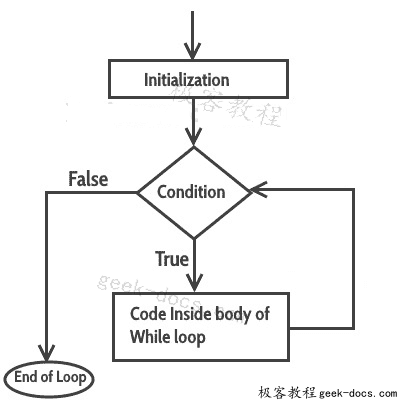# C语言 while循环

C语言 while循环，循环用于重复执行语句块，直到给定条件返回false。在上一个教程中，我们学习了C语言 for循环。在本指南中，我们将学习 while循环

## C while循环

while循环的语法：

while (condition test)
{
//Statements to be executed repeatedly
// Increment (++) or Decrement (--) Operation
}


### while 循环流程图### while循环的示例

#include <stdio.h>
int main()
{
int count=1;
while (count <= 4)
{
printf("%d ", count);
count++;
}
return 0;
}


1 2 3 4


#include <stdio.h>
int main()
{
int var=1;
while (var <=2)
{
printf("%d ", var);
}
}


### 无限while循环的例子

#include <stdio.h>
int main()
{
int var = 6;
while (var >=5)
{
printf("%d", var);
var++;
}
return 0;
}


#include <stdio.h>
int main()
{
int var =5;
while (var <=10)
{
printf("%d", var);
var--;
}
return 0;
}


## 在while循环中使用逻辑运算符

while(num1<=10 && num2<=10)


while(num1<=10||num2<=10)


while(num1!=num2 &&num1 <=num2)


while(num1!=10 ||num2>=num1)


### 使用逻辑运算符的while循环示例

#include <stdio.h>
int main()
{
int i=1, j=1;
while (i <= 4 || j <= 3)
{
printf("%d %d\n",i, j);
i++;
j++;
}
return 0;
}


1 1
2 2
3 3
4 4


• 回顶